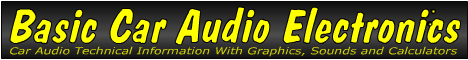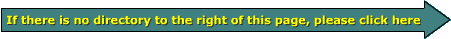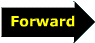Volt

Previously, you saw how a high water column or a higher stack of cells could produce more pressure/voltage. In the following image, you can see how the lower pressure at the higher pipe produces less 'current' flow than the lower one (which has a greater force behind it due to the higher water pressure).

In the following image, the batteries are driving fans. Each individual battery is driving a fan with the 6v it produces. the left-most fan is connected across both batteries. It's spinning twice as fast because it's receiving twice the voltage. Of course, in the real world, the fan with twice as much voltage wouldn't spin precisely twice as fast but it would spin much faster than the other two fans. What's important here is to see that doubling the voltage across the a given load does more work.

Now for a more technical definition. 1 volt of electrical pressure will, when connected to a load resistance of 1 ohms, drive 1 amp of current through that 1 ohm load. Driving 2 volts into that same 1 ohm load, will force twice the electrical current through the 1 ohm load.

 You should remember: 1.The volt is the unit of measure for electrical force (pressure).```

```